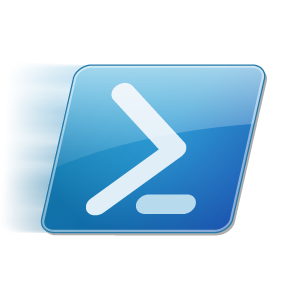With this script you will be able to generate a random password with custom character sets :

• define a set for the lower case characters : variable \$chars_low
• define a set for the upper case characters : variable \$chars_upp
• define a set for the numbers : variable \$chars_num
• define a set for the special characters : variable \$chars_spc

You can define also how many characters you want to insert in the final password in each set (variables \$x_low, \$x_upp, \$x_num and \$x_spc)

In the following example, a random password is generated with these following rules :

• the password will contain 5 lower case characters
• the password will contain 5 upper case characters
• the password will contain 5 numbers
• the password will contain 2 special characters
```\$chars_low = [Char[]]"abcdefghijklmnopqrstuvwxyz"
\$chars_upp = [Char[]]"ABCDEFGHIJKLMNOPQRSTUVWXYZ"
\$chars_num = [Char[]]"0123456789"
\$chars_spc = [Char[]]".-_+,"
\$x_low = 5
\$x_upp = 5
\$x_num = 5
\$x_spc = 2
\$x_tot = \$x_low + \$x_upp + \$x_num + \$x_spc

\$TempPassword=	(\$chars_low | Get-Random -Count \$x_low)+ `
(\$chars_upp | Get-Random -Count \$x_upp)+ `
(\$chars_num | Get-Random -Count \$x_num)+ `
(\$chars_spc | Get-Random -Count \$x_spc)

(\$TempPassword | Get-Random -Count \$x_tot) -join ""
```
<>

My Powershell script categories

Generate a random password with custom character sets